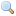# Module type Weak.S

`module type S = `sig` .. `end``
The output signature of the functor `Weak.Make`.

`type data `
The type of the elements stored in the table.
`type t `
The type of tables that contain elements of type `data`. Note that weak hash tables cannot be marshaled using `Pervasives.output_value` or the functions of the `Marshal` module.
`val create : `int -> t``
`create n` creates a new empty weak hash table, of initial size `n`. The table will grow as needed.
`val clear : `t -> unit``
Remove all elements from the table.
`val merge : `t -> data -> data``
`merge t x` returns an instance of `x` found in `t` if any, or else adds `x` to `t` and return `x`.
`val add : `t -> data -> unit``
`add t x` adds `x` to `t`. If there is already an instance of `x` in `t`, it is unspecified which one will be returned by subsequent calls to `find` and `merge`.
`val remove : `t -> data -> unit``
`remove t x` removes from `t` one instance of `x`. Does nothing if there is no instance of `x` in `t`.
`val find : `t -> data -> data``
`find t x` returns an instance of `x` found in `t`. Raise `Not_found` if there is no such element.
`val find_all : `t -> data -> data list``
`find_all t x` returns a list of all the instances of `x` found in `t`.
`val mem : `t -> data -> bool``
`mem t x` returns `true` if there is at least one instance of `x` in `t`, false otherwise.
`val iter : `(data -> unit) -> t -> unit``
`iter f t` calls `f` on each element of `t`, in some unspecified order. It is not specified what happens if `f` tries to change `t` itself.
`val fold : `(data -> 'a -> 'a) -> t -> 'a -> 'a``
`fold f t init` computes `(f d1 (... (f dN init)))` where `d1 ... dN` are the elements of `t` in some unspecified order. It is not specified what happens if `f` tries to change `t` itself.
`val count : `t -> int``
Count the number of elements in the table. `count t` gives the same result as `fold (fun _ n -> n+1) t 0` but does not delay the deallocation of the dead elements.
`val stats : `t -> int * int * int * int * int * int``
Return statistics on the table. The numbers are, in order: table length, number of entries, sum of bucket lengths, smallest bucket length, median bucket length, biggest bucket length.